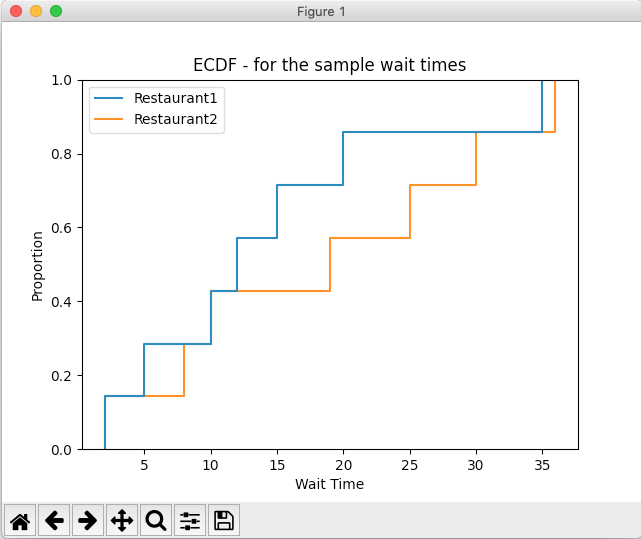# Drawing Empirical Cumulative Distribution Function(ECDF) Using Seaborn

## Overview:

• A Cumulative Distribution Function(CDF) returns the probabilities of a range of outcomes for a random variable either discrete or continuous.
• When the Cumulative Distribution Function describes probabilities of sample outcomes drawn from a population, it is called the Empirical Cumulative Distribution Function(ECDF).
• ECDF answers the following questions:
• What is the probability that outcome of a random variable(sample value from the population) is less than or equal to a certain value? The answer is to sum the probabilities of each individual outcome.
• The Empirical Cumulative Probability Distribution function P(X) is given by

P(X)=p(x1)+p(x2)+⋯p(xn)

where x1, x2, xn-1 ≤ xn.

X – name of the random variable

x – value of the random variable or outcome from the sample

• In an ECDF plot, the Y axis denotes the probabilities. It also denotes the percentile values of the sample. For example, 0.2 in the Y axis refers to the 20th percentile of the probabilities. Correlating with the X axis one can get the individual probability of a certain outcome, as well as the cumulative probability of the outcome being less than or equal to that value.
• The function ecdfplot() of the seaborn library draws the ECDF plot for a given sample data.

## Example:

 # Example Python program that plots the  # Empirical Cumulative Distribution Function(ECDF) # for a sample of values drawn from a population import seaborn as sns import matplotlib.pyplot as plt # Restaurants and their Sample wait times d1 = {"Restaurant1":[2, 5, 10, 12, 15, 20, 35],       "Restaurant2":[2, 8, 10, 19, 25, 30, 36]}; # Draw the ECDF plot (a.k.a, Step Plot) sns.ecdfplot(data=d1); plt.xlabel('Wait Time'); plt.title("ECDF - for the sample wait times"); plt.show();

## Output: